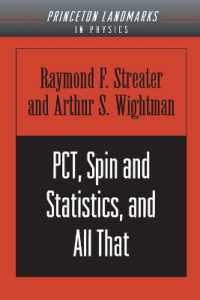### PCT, Spin and Statistics, and All That (Princeton Landmarks in Mathematics and Physics)

• 提携先の海外書籍取次会社に在庫がございます。通常3週間で発送いたします。
重要ご説明事項
1. 納期遅延や、ご入手不能となる場合が若干ございます。
2. 複数冊ご注文の場合、分割発送となる場合がございます。
3. 美品のご指定は承りかねます。
• 【重要：入荷遅延について】
ウクライナ情勢悪化・新型コロナウィルス感染拡大により、洋書・洋古書の入荷が不安定になっています。詳しくはこちらをご確認ください。
海外からのお取り寄せの場合、弊社サイト内で表示している標準的な納期よりもお届けまでに日数がかかる見込みでございます。
申し訳ございませんが、あらかじめご了承くださいますようお願い申し上げます。
• ◆画像の表紙や帯等は実物とは異なる場合があります。
• 製本 Paperback:紙装版/ペーパーバック版／ページ数 224 p.
• 言語 ENG
• 商品コード 9780691070629
• DDC分類 530.143

### Full Description

PCT, Spin and Statistics, and All That is the classic summary of and introduction to the achievements of Axiomatic Quantum Field Theory. This theory gives precise mathematical responses to questions like: What is a quantized field? What are the physically indispensable attributes of a quantized field? Furthermore, Axiomatic Field Theory shows that a number of physically important predictions of quantum field theory are mathematical consequences of the axioms. Here Raymond Streater and Arthur Wightman treat only results that can be rigorously proved, and these are presented in an elegant style that makes them available to a broad range of physics and theoretical mathematics.

### Contents

*Frontmatter, pg. i*Contents, pg. v*Preface, pg. vii*Introduction, pg. 1*1. Relativistic Transformation Laws, pg. 4*2. Some Mathematical Tools, pg. 31*3. Fields and Vacuum Expectation Values, pg. 96*4. Some General Theorems of Relativistic Quantum Field Theory, pg. 134*Appendix, pg. 179*Index, pg. 205